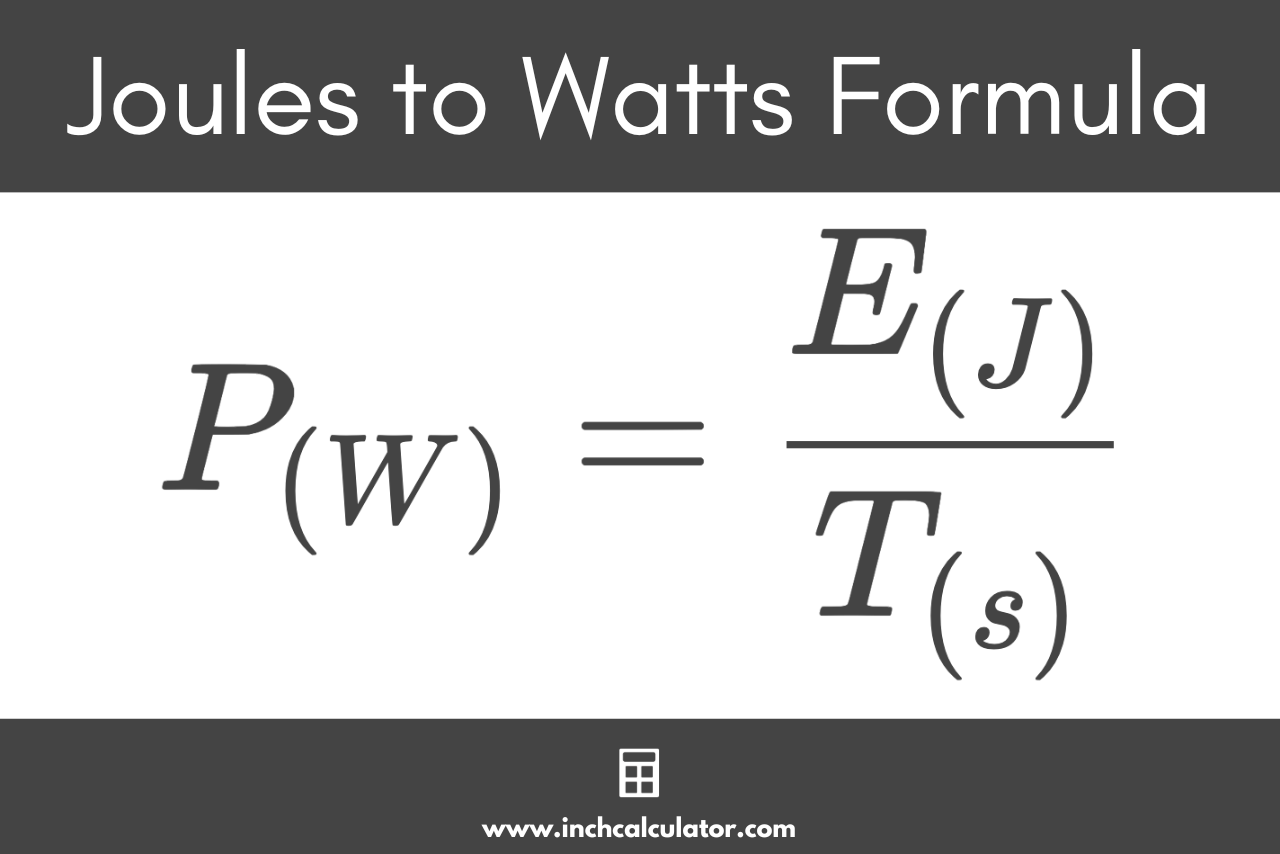# Joules to Watts Conversion Calculator

Convert joules to watts by entering the energy and time in seconds below.

joules
seconds

## Power:

watts
Learn how we calculated this below

## How to Convert Joules to Watts

Before we go over how to convert joules to watts, we need to cover some basic terminology that we’ll be using in the formula below.

Energy is the ability of a system to perform work and is measured in joules (J). One joule is equal to the amount of energy dissipated to move a 1-kilogram object 1 meter using a constant force of 1 newton.

Power is calculated to measure the rate at which work is done on a system and measured in watts (W). One watt of power equals the rate at which a 1 joule of energy is delivered in 1 second.

For example, a light bulb rated at 100 watts will consume energy at a rate of 100 joules/second.

### Joules to Watts Formula

If you know the amount of energy dissipated over a period of time in seconds, you can use this formula to convert joules to watts:

P(W) = E(J) / T(s)

The power P in watts is equal to the energy E in joules divided by the time T in seconds.

Substitute joules and time in seconds in the formula above to convert to watts.Do you want to convert watts to joules?

For example, let’s find the power consumed in watts when a device uses 250 joules of energy over a time period of 40 seconds:

P(W) = 250 / 40
P(W) = 6.25

You might also like our watts to amps converter for converting power to current.